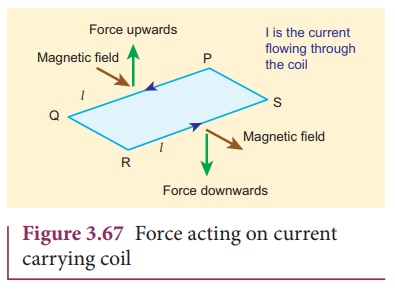Home | | Physics 12th Std | Moving coil galvanometer

# Moving coil galvanometer

Moving coil galvanometer is a device which is used to indicate the flow of current in an electrical circuit.

Moving coil galvanometer

Moving coil galvanometer is a device which is used to indicate the flow of current in an electrical circuit.

## Principle

When a current carrying loop is placed in a uniform magnetic field it experiences a torque.

## Construction

A moving coil galvanometer consists of a rectangular coil PQRS of insulated thin copper wire. The coil contains a large number of turns wound over a light metallic frame. A cylindrical soft-iron core is placed symmetrically inside the coil as shown in Figure 3.66. The rectangular coil is suspended freely between two pole pieces of a horse-shoe magnet.The upper end of the rectangular coil is attached to one end of fine strip of phosphor bronze and the lower end of the coil is connected to a hair spring which is also made up of phosphor bronze. In a fine suspension strip W, a small plane mirror is attached in order to measure the deflection of the coil with the help of lamp and scale arrangement. The other end of the mirror is connected to a torsion head T. In order to pass electric current through the galvanometer, the suspension strip W and the spring S are connected to terminals.

## Working

Consider a single turn of the rectangular coil PQRS whose length be l and breadth b. PQ = RS = l and QR = SP = b. Let I be the electric current flowing through the rectangular coil PQRS as shown in Figure 3.67.The horse-shoe magnet has hemi - spherical magnetic poles which produces a radial magnetic field. Due to this radial field, the sides QR and SP are always parallel to to the B-field (magnetic field) and experience no force. The sides PQ and RS are always parallel to the B-field and experience force and due to this, torque is produced.For single turn, the deflection couple as shown in Figure 3.68 is

τ = bF = bBIl= (lb) BI = ABI

since, area of the coil A = lb

For coil with N turns, we getDue to this deflecting torque, the coil gets twisted and restoring torque (also known as restoring couple) is developed. Hence the magnitude of restoring couple is proportional to the amount of twist θ (Refer Unit 10 of Std. XI Physics).Thuswhere K is the restoring couple per unit twist or torsional constant of the spring.

At equilibrium, the deflection couple is equal to the restoring couple. Therefore by comparing equation (3.69) and (3.70), we get(or) I = G θ

where, G = K/NAB = is called galvanometer constant or current reduction factor of the galvanometer.

Since, suspended moving coil galvanometer is very sensitive, we have to handle with high care while doing experiments. Most of the galvanometer we use are pointer type moving coil galvanometer.

### Figure of merit of a galvanometer

It is defined as the current which produces a deflection of one scale division in the galvanometer.

## Sensitivity of a galvanometer

The galvanometer is said to be sensitive if it shows large scale deflection even though a small current is passed through it or a small voltage is applied across it.

Current sensitivity: It is defined as the deflection produced per unit current flowing through it.The current sensitivity of a galvanometer can be increased

(a) by increasing

(1) the number of turns N

(2) the magnetic induction B

(3) the area of the coil A

(b) by decreasingthe couple per unit twist of the suspension wire k. Phosphor - bronze wire is used as the suspension wire because the couple per unit twist is very small.

Voltage sensitivity: It is defined as the deflection produced per unit voltage applied across it.where Rg is the resistance of galvanometer.

EXAMPLE 3.29

The coil of a moving coil galvanometer has 5 turns and each turn has an effective area of 2 × 10-2 m2. It is suspended in a magnetic field whose strength is 4 × 10-2 Wb m-2. If the torsional constant K of the suspension fibre is 4 × 10-9 N m deg-1.

(a) Find its current sensitivity in degree per micro - ampere.

(b) Calculate the voltage sensitivity of the galvanometer for it to have full scale deflection of 50 divisions for 25 mV.

(c) Compute the resistance of the galvanometer.

Solution

The number of turns of the coil is 5 turns

The area of each coil is 2 × 10-2 m2

Strength of the magnetic field is 4 × 10-2 Wb m-2

Torsional constant is 4 × 10-9 N m deg-1EXAMPLE 3.30

The resistance of a moving coil galvanometer is made twice its original value in order to increase current sensitivity by 50%. Will the voltage sensitivity change? If so, by how much?.

Solution

Yes, voltage sensitivity will change.

Voltage sensitivity is Vs = Is/R

When the resistance is doubled, then new resistance is R ′ = 2R

Increase in current sensitivity isHence the voltage sensitivity decreases. The percentage decrease in voltage sensitivity isTags : Principle, Construction, Working Principle, Sensitivity, Solved Example Problems , 12th Physics : Magnetism and Magnetic Effects of Electric Current
Study Material, Lecturing Notes, Assignment, Reference, Wiki description explanation, brief detail
12th Physics : Magnetism and Magnetic Effects of Electric Current : Moving coil galvanometer | Principle, Construction, Working Principle, Sensitivity, Solved Example Problems